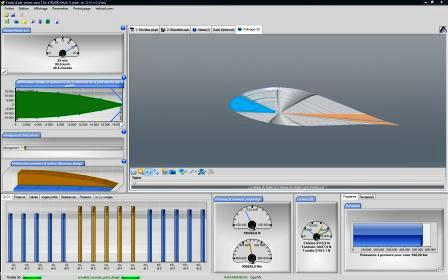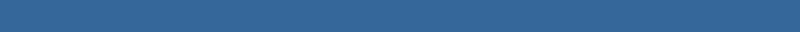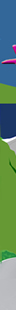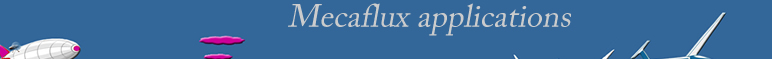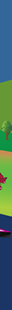See also:

• Forces in Newtons
• Density in kg/m3
• Surface in m²
• Coefficients as Cv , Re, Fr have no units

the displacement of a vessel is the weight of water it displaces, so its volume immersed X density of water.

HYDRODYNAMICSHydrodynamics, general

When an object is immersed in a fluid stream, there is phenomena of friction and turbulence.

• when the fluid is air, the study of these phenomena is Aerodynamics.
• when the fluid is water, the study of these phenomena is Hydrodynamics.

The study of hydrodynamic resistance (Rh) does not currently have a comprehensive theory that would apply an equation corresponding to different cases accurately. We therefore divided the phenomena within certain parameters:

• If the object moves completely immersed (see: Drag)  the hydrodynamic coefficient will be studied by combining the frictional resistance to the resistance form. The combination of these two resistances is called the viscous resistance or drag. The viscous resistance Rv (Newtons) or drag , whose coefficient of viscous resistance is (Cv or Cd if the drag has been measured in wind tunnel) is related to the Reynolds number (Re) and the roughness (K) relating to the hull length (L)
• Rv= Cv . (Re . K/L)
• Drag= Cd .r.s.(v²/2) Cd is relating to Reynolds number, the extrapolation to the hydrodynamics is possible..

The total coefficient of hydrodynamic resistance (Ch=Rh/displacement) for an object in the deep sea without surface effect is defined as:

• Ch = Rv= Cv.(Re.K/L)

• If the object moves near the surface (naval hydrodynamics) viscous resistance or drag is accompanied by a resistance due to the formation of surface waves, the wave resistance (Rw), whose coefficient of wave resistance (Cw) is related to the Froude_number_Fr as:
• Rw= Cw.(Fr)
• Cw =Rw/(1/2)r.s.v²(r water density, S wet surface, V velocity.

The total coefficient of hydrodynamic resistance Ch = Rh / displacement for an object moving close to the surface is defined as:

Ch=  Cw.(Fr) + Cv.(Re . K/L)

• If this object is shaped to "Fly" (foils hydrofoils) in water, we can make a study of classical aerodynamic lift and drag. Cd and CL (drag and lift coefficients) are given according to Reynolds numbers, the extrapolation to the hydrodynamics is therefore possible.

• If this object is a kind of hovercraft (see propellers) hydrodynamic resistance forces will be significantly reduced, but we need to quantify the energy expended to create air pressure.

Different types of approaches used to evaluate the hydrodynamic coefficient (Ch):

Despite advances in computer modeling, testing models are still essential.The effects of friction measured experimentally basins hulls, are themselves subject to scaling problems, solved by the laws of similarities (See: Froude)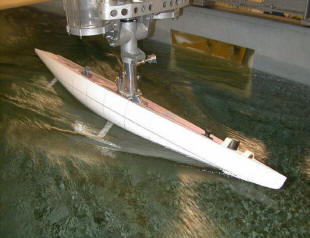testing model Towing tank

Another technique for evaluating hydrodynamic behavior is to compare measured data from a series of standard forms and extrapolate the consequences of changing forms.

 serie Taylor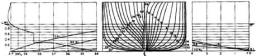serie NPL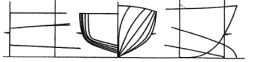serie Nordstrom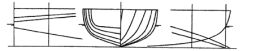63 serie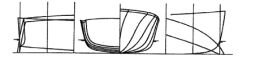serie SSPA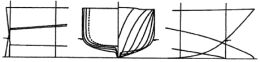64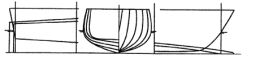The naval hydrodynamics studied resistance to the advancement of ships, en savoir plus sur l'hydrodynamique navale

See also: